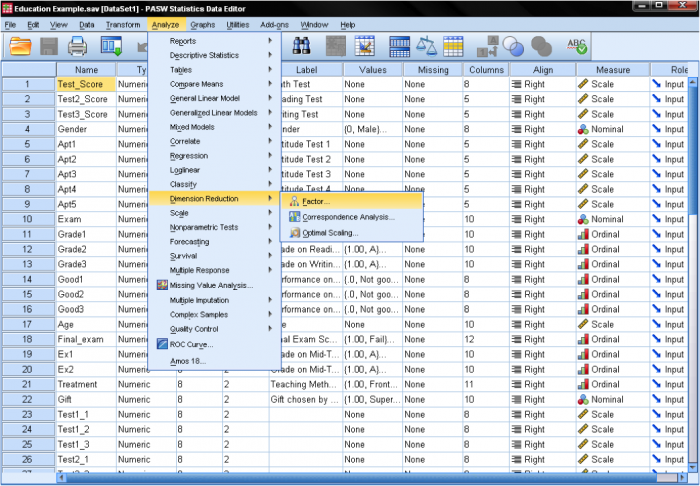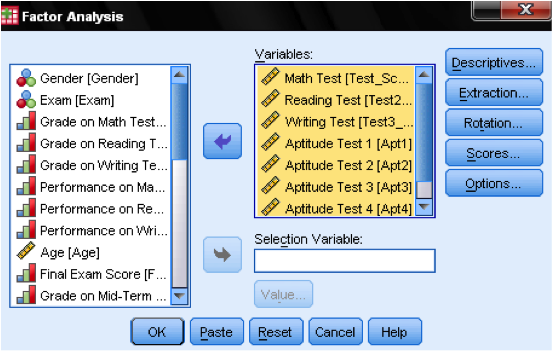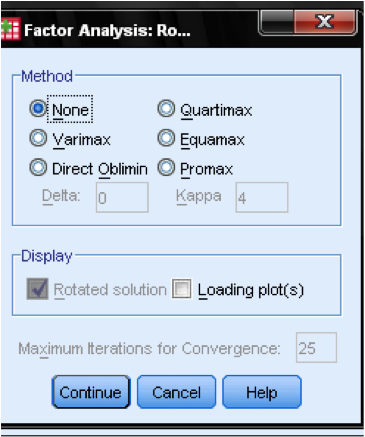# Conduct and Interpret a Factor Analysis

What is the Factor Analysis?

Much like cluster analysis involves grouping similar cases, factor analysis involves grouping similar variables into dimensions.  This process is used to identify latent variables or constructs.  The purpose of factor analysis is to reduce many individual items into a fewer number of dimensions.  Factor analysis can be used to simplify data, such as reducing the number of variables in regression models.

Most often, factors are rotated after extraction.  Factor analysis has several different rotation methods, and some of them ensure that the factors are orthogonal (i.e., uncorrelated), which eliminates problems of multicollinearity in regression analysis.Factor analysis is also used to verify scale construction.  In such applications, the items that make up each dimension are specified upfront.  This form of factor analysis is most often used in the context of structural equation modeling and is referred to as confirmatory factor analysis.  For example, a confirmatory factor analysis could be performed if a researcher wanted to validate the factor structure of the Big Five personality traits using the Big Five Inventory.

Factor analysis can also be used to construct indices.  The most common way to construct an index is to simply sum up all the items in an index.  However, some variables that make up the index might have a greater explanatory power than others.  A factor analysis could be used to justify dropping questions to shorten questionnaires.

The Factor Analysis in SPSS

The research question we want to answer with our exploratory factor analysis is:

What are the underlying dimensions of our standardized and aptitude test scores?   That is, how do aptitude and standardized tests form performance dimensions?

The factor analysis can be found in Analyze/Dimension Reduction/Factor…In the dialog box of the factor analysis we start by adding our variables (the standardized tests math, reading, and writing, as well as the aptitude tests 1-5) to the list of variables.In the dialog Descriptives… we need to add a few statistics to verify the assumptions made by the factor analysis.  To verify the assumptions, we need the KMO test of sphericity and the Anti-Image Correlation matrix.The dialog box Extraction… allows us to specify the extraction method and the cut-off value for the extraction.  Generally, SPSS can extract as many factors as we have variables.  In an exploratory analysis, the eigenvalue is calculated for each factor extracted and can be used to determine the number of factors to extract.  A cutoff value of 1 is generally used to determine factors based on eigenvalues.Next, an appropriate extraction method need to be selected.  Principal components is the default extraction method in SPSS.  It extracts uncorrelated linear combinations of the variables and gives the first factor maximum amount of explained variance.  All following factors explain smaller and smaller portions of the variance and are all uncorrelated with each other.  This method is appropriate when the goal is to reduce the data, but is not appropriate when the goal is to identify latent constructs.

The second most common extraction method is principal axis factoring.  This method is appropriate when attempting to identify latent constructs, rather than simply reducing the data. In our research question, we are interested in the dimensions behind the variables, and therefore we are going to use principal axis factoring.

The next step is to select a rotation method.  After extracting the factors, SPSS can rotate the factors to better fit the data.  The most commonly used method is varimaxVarimax is an orthogonal rotation method that tends produce factor loading that are either very high or very low, making it easier to match each item with a single factor.  If non-orthogonal factors are desired (i.e., factors that can be correlated), a direct oblimin rotation is appropriate.  Here, we choose varimax.In the dialog box Options we can manage how missing values are treated – it might be appropriate to replace them with the mean, which does not change the correlation matrix but ensures that we do not over penalize missing values.  Also, we can specify in the output if we do not want to display all factor loadings.  The factor loading tables are much easier to read when we suppress small factor loadings.  Default value is 0.1, but in this case, we will increase this value to 0.4.  The last step would be to save the results in the Scores… dialog.  This automatically creates standardized scores representing each extracted factor.